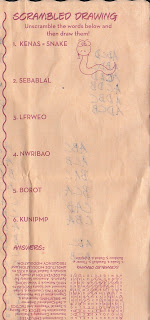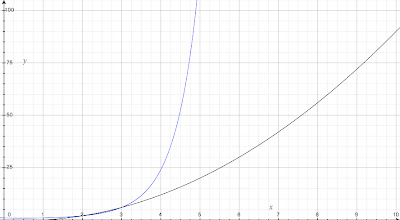## 9.08.2009

### Svilnog aagrnams

Last Saturday I was sitting at Monical's Pizza, scarfing down a bowl of the red and white salad dressings with some lettuce, when I began pondering the anagrams on my placemat.I managed to quickly solve the first one, then stumbled over the next three and picked up the last two. For the rest of the salad I remained stumped, unable to fathom the intended sequences.

Unfortunately this is just the sort of luck I have with anagrams; my brain simply refuses to make sense of them. I'm sure I would improve if I made it a point to solve an anagram or two each day, but I've never had the discipline to do so.

After the pizza arrived I began thinking about how humans are able to easily read words whose letters have been jumbled, assuming the first and last letters are correct. If one were only concerned about getting the first and last letters right, then the number of possible combinations one might have to consider would be greatly reduced.

Consider a three-letter anagram:

If you were only concerned with the number of possible combinations for the first and last letters, then you might come up with a list like this:

AxB
AxD
BxA
BxD
DxA
DxB

However there would be no inherent advantage to this as the number of combinations using all three letters is exactly the same:

ABD
BDA
DBA
DAB

The number of unique combinations for the letters in a given word can be expressed as x! (factorial of x). Here, using three letters, we have 3 x 2 x 1 = 6, or 3!, unique combinations for the order of the letters. Again, focusing on the first and last letters here doesn't help much. It's when we examine the differences in a word with four letters that we begin to see the potential.

RFOU OFUR
RFUO OFRU
RUOF ORFU
RUFO ORUF
ROFU OURF
ROUF OUFR
UFOR FURO
UFRO FUOR
UROF FROU
URFO FRUO
UOFR FORU
UORF FOUR

Here, there are 4 x 3 x 2 x 1 = 24, or 4!, unique combinations. But if we focus only on the combinations for the first and last letters, we could cut this number in half.

UxxO
UxxR
UxxF
OxxR
OxxF
OxxU
RxxO
RxxU
RxxF
FxxO
FxxU
FxxR

The number of combinations for the first and last letters can be expressed as x · (x - 1) or x2- x. The advantage of this method is best viewed as the difference of two curves.Here, the blue curve is the graph of the function y = x! and the black curve is the graph of the function y = x2- x where x is the number of letters and y is the number of combinations. Notice that, just as in the example, the number of combinations is the same until more than three letters are encountered, at which point the advantage can be seen. Indeed there is an enormous advantage with anagrams as short as five letters.

With such a simple method for attacking future anagrams, it was only right that I slap together a BASH script to put this method into practice.
``````#!/bin/bash

anagram=( \$(echo \$1 | sed -e 's/\(.\)/\1 /g') )

for ((i = 0; i<\${#anagram[@]}; i++)); do
copy=( \${anagram[@]} )
unset copy[\$i]
copy=( \${copy[@]} )
for ((j = 0; j<\${#copy[@]}; j++)); do
copy2=( \${copy[@]} )
unset copy2[\$j]
copy2=`echo \${copy2[@]} | sed -e 's/ //g'`
echo \${anagram[\$i]}\$copy2\${copy[\$j]}
done
````done`
```

Providing an anagram as the only argument, this script will produce a list containing all possible combinations for the first and last letters without changing the letters in the middle. In the short amount of time I've spent playing with this I've found it to be quite useful. That is, I've found it to be as useful as a helper script for solving anagrams probably can be. Useful ideas aren't nearly as fun, anyway.

EDIT: A real anagram solver can be found here: http://www.ssynth.co.uk/~gay/anagram.html.

09/09/2009 - EDIT: I acknowledge that this is almost entirely limited to "scrambled-word" anagrams. While you could probably use this with actual anagrams, you would of course be limited to solving one-word combinations.

. . .